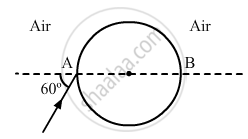Share

# A Ray of Light Falls on a Transparent Sphere with Centre C as Shown in the Figure. the Ray Emerges from the Sphere Parallel to the Line Ab. - Physics

ConceptThe Parallel Plate Capacitor

#### Question

A ray of light falls on a transparent sphere with centre C as shown in the figure. The ray emerges from the sphere parallel to the line AB. Find the angle of refraction at A if the refractive index of the material of the sphere is $\sqrt{3}$.#### Solution

From Snell's law, we have: $\frac{\sin\left( i \right)}{\sin\left( r \right)} = \mu$ At A, i = 60° (given)
Now, μ = √3

$\Rightarrow \sin\left( r \right) = \frac{\sin\left( i \right)}{\mu}$

$\Rightarrow \sin\left( r \right) = \frac{\sin\left( 60° \right)}{\sqrt{3}} = \frac{1}{2}$

$\Rightarrow r = \sin^{- 1} \left( \frac{1}{2} \right)$

$\therefore r = 30°$

Is there an error in this question or solution?

#### APPEARS IN

Solution A Ray of Light Falls on a Transparent Sphere with Centre C as Shown in the Figure. the Ray Emerges from the Sphere Parallel to the Line Ab. Concept: The Parallel Plate Capacitor.
S